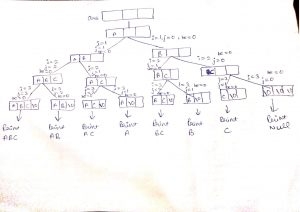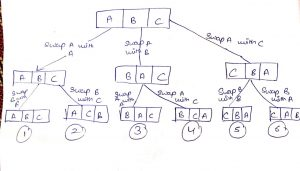Create all possible strings from a given set of characters in c++

In this tutorial, we are going to learn how to print all possible strings of any length from a given string or a given set of characters in C++. We can also modify our code by keeping a length constraint for the length of strings. For example :

If a given string “abc” then the possible strings of any length will be:

abc acb bac bca cba cab ab ba ac ca a bc cb b c

But in case we want only strings of length 2, we can modify our code also with it.

Create all possible strings from a given set of characters in C++

So initially the approach to this will be divided into two steps:

1. Finding subsets of the given string
2. Finding permutations of all the given subset one by one

And we could find all the possible strings of all lengths.

We will be finding the subsets and permutation of a string by recursion.

The way to find subsets of a given string is explained in this image with the help of a recursion treesubset recursion tree

and to find the permutation of a given string can be explained from this recursion treepermutation recursion tree

The code is here:

#include<bits/stdc++.h>
using namespace std;

void printPermutations(char arr[], int be){
if (arr[be] == '\0')//base case
{
cout  << arr<<" " ;
return;
}

for(int pos = be; arr[pos] != '\0'; ++pos)
{
swap(arr[pos], arr[be]);
printPermutations(arr, be + 1);//recursive call
swap(arr[pos], arr[be]);
}
}

void subsets(char in[],char ans[],int i,int j,int be)
{
if(in[i]=='\0')//if we reached at the end of our input array-base case:then print the subset
{
ans[j]='\0';//terminate the temp array this is the required subset
printPermutations(ans, 0);//calling permutation function to find all the permutations of the given subset
return;
}

ans[j]=in[i];//else if we have not reached to the end of input array
subsets(in,ans,i+1,j+1,be);//increase both i and j
subsets(in,ans,i+1,j,be);//inc only i

}

int main()
{
char input="abcd"; //creating input array

char ans;//creating a temp array which will store the subsets

subsets(input,ans,0,0,0);//this will compute all the subsets of the given string
}

The output will be:

1.Archita Yadav says: